# 8 Raster Spatial Analysis

Raster spatial analysis is particularly important in environmental analysis, since much environmental data are continuous in nature, based on continuous measurements from instruments (like temperature, pH, air pressure, water depth, elevation, to name a few.) In the “Spatial Data and Maps” chapter, we looked at creating rasters from scratch and visualizing them. Here we’ll explore raster analytical methods, commonly working from existing information-rich rasters like elevation data, where we’ll start by looking at terrain functions.

We’ll make a lot of use of terra functions in this chapter, as this package is replacing the raster package which has been widely used. One raster package that’s probably also worth considering is the stars package.

## 8.1 Terrain functions

Elevation data are particularly information-rich, and a lot can be derived from them that informs us about the nature of landscapes and what drives surface hydrologic and geomorphic processes as well as biotic habitat (some slopes are drier than others, for instance). We’ll start by reading in some elevation data from the Marble Mountains of California and use terra’s terrain function to derive slope, aspect, and hillshade rasters…

library(terra); library(igisci)
elev <- rast(ex("marbles/elev.tif"))
plot(elev)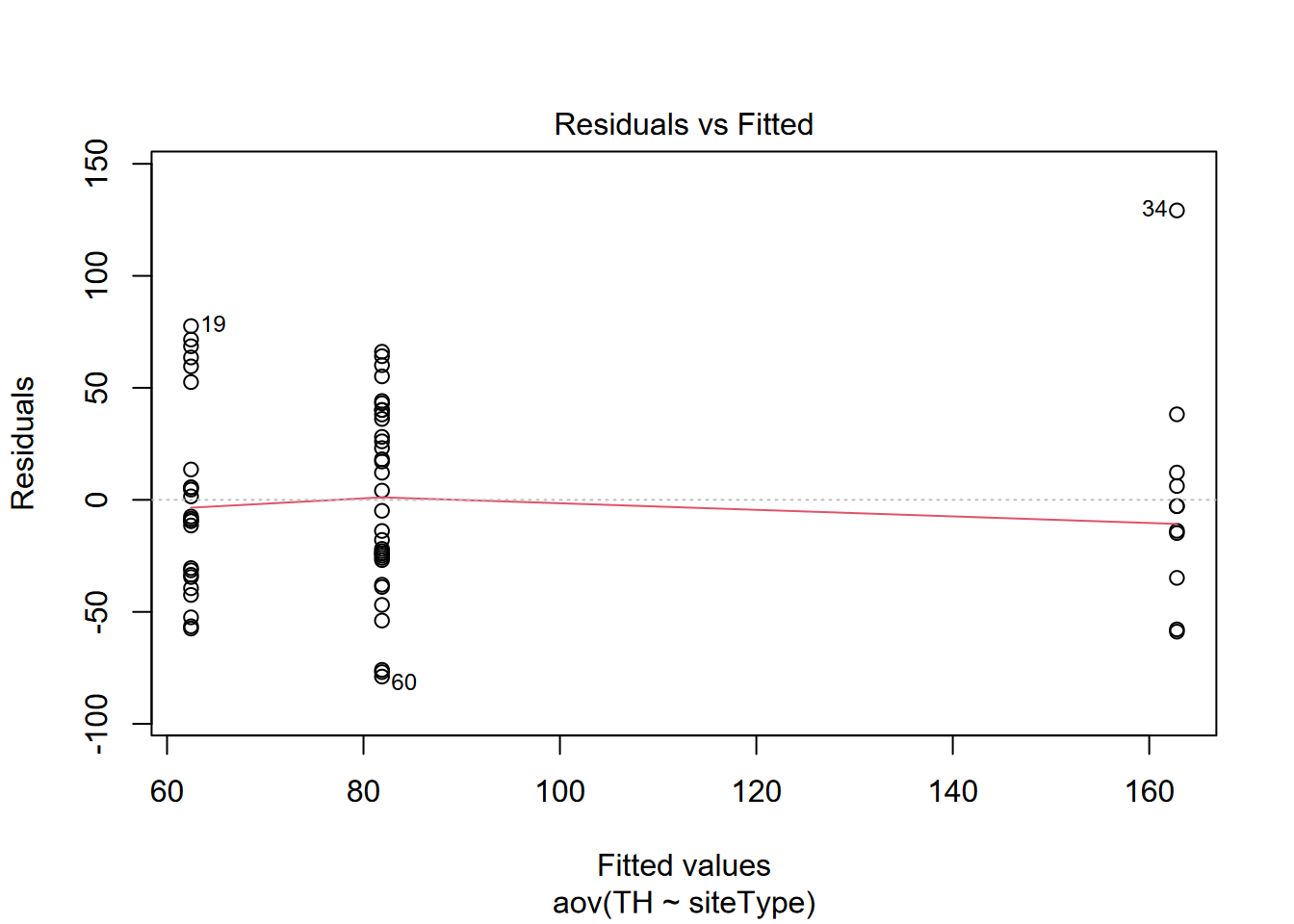Figure 8.1: Marbles elevation

slope <- terrain(elev, v="slope")
plot(slope)Figure 8.2: Slope

aspect <- terrain(elev, v="aspect")
plot(aspect)Figure 8.3: Aspect

… then we’ll use terra::classify to make six discrete categorical slope classes (though the legend suggests it’s continuous)…

slopeclasses <-matrix(c(00,10,1, 10,20,2, 20,30,3,
30,40,4, 40,50,5, 50,90,6), ncol=3, byrow=TRUE)
slopeclass <- classify(slope, rcl = slopeclasses)
plot(slopeclass)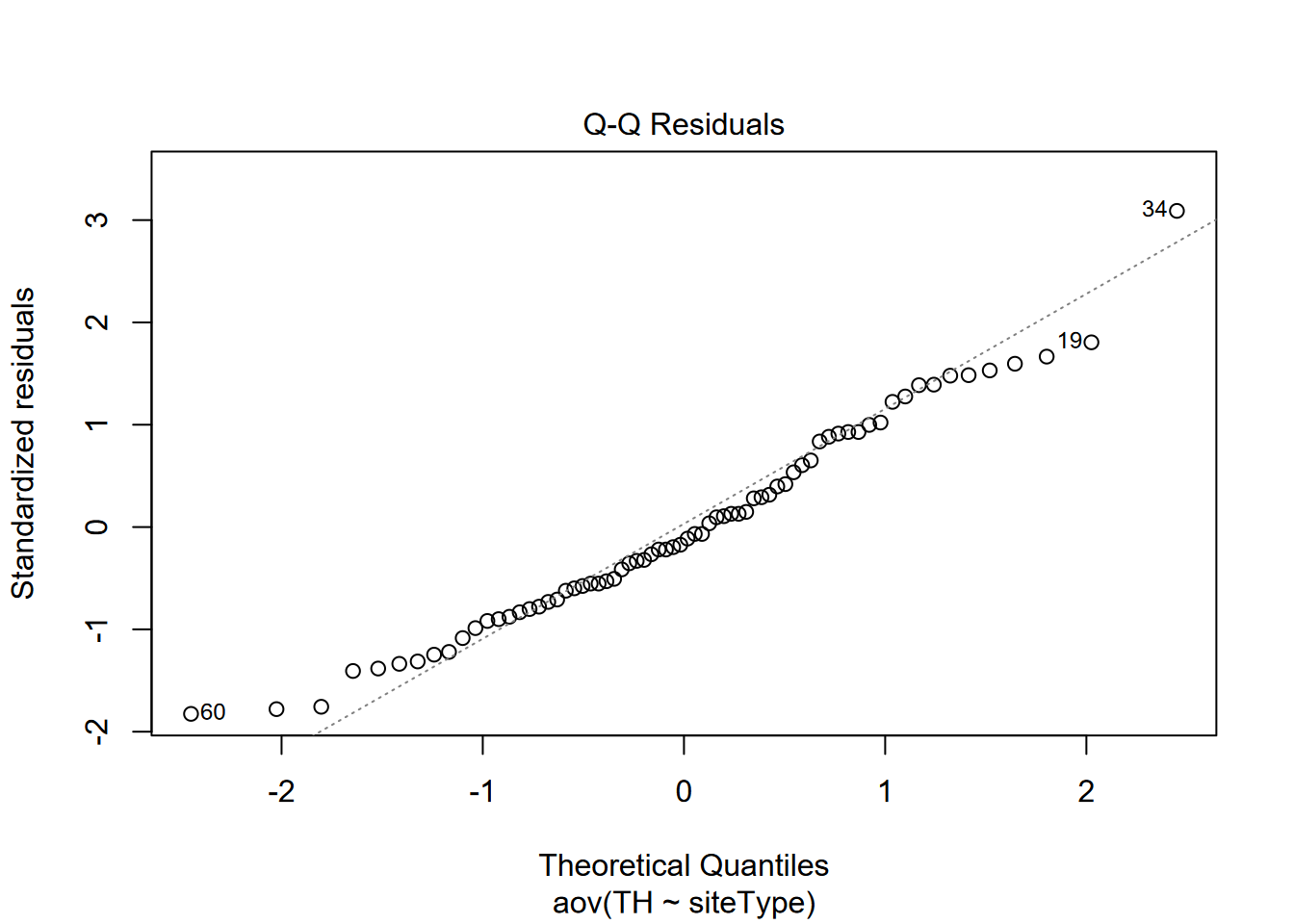Figure 8.4: Classified slopes

Then a hillshade effect raster with slope and aspect as inputs after converting to radians:

hillsh <- shade(slope/180*pi, aspect/180*pi, angle=40, direction=330)
plot(hillsh, col=gray(0:100/100))## 8.2 Map algebra in terra

Map algebra was originally developed by Dana Tomlin in the 1970s and 1980s (), and was the basis for his Map Analysis Package. It works by assigning raster outputs from an algebraic expression of raster inputs. Map algebra was later incorporated in Esri’s Grid and Spatial Analyst subsystems of ArcInfo and ArcGIS. Its simple and elegant syntax makes it still one of the best ways to manipulate raster data.

Let’s look at a couple of simple map algebra statements to derive some new rasters …

elevft <- elev / 0.3048
plot(elevft)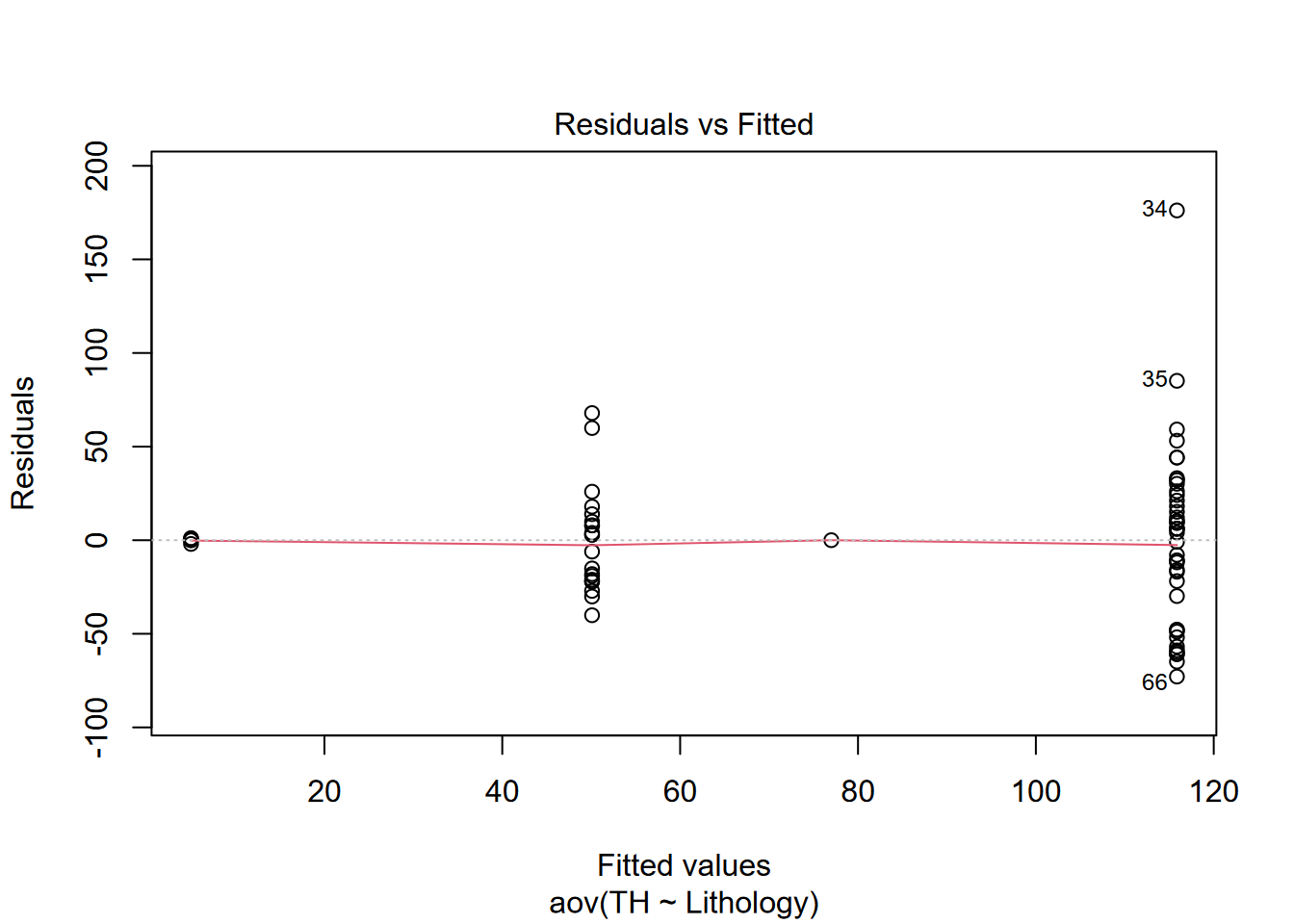Figure 8.6: Map algebra conversion of elevations from metres to feet

… including some that create and use Boolean (true-false) values where 1 is true and 0 is false, so answers the question “Is it steep?” (as long as we understand 1 means Yes or true) …

steep <- slope > 20
plot(steep)Figure 8.7: Boolean: slope > 20

… or this map that shows all areas that are steeper than 20 degrees and above 2000 m elevation:

plot(steep * (elev > 2000))Figure 8.8: Boolean intersection: (slope > 20) * (elev > 2000)

You should be able to imagine that map algebra is particularly useful when applying a model equation to data to create a prediction map. For instance, later we’ll use lm() to derive linear regression parameters for predicting February temperature from elevation in the Sierra …

$Temperature_{prediction} = 11.88 - 0.006{elevation}$

… which can be coded in map algebra something like the following if we have an elevation raster, to create a tempPred raster:

tempPred <- 11.88 - 0.006 * elevation

## 8.3 Distance (and vector-raster conversion)

A continuous raster of distances from significant features can be very informative in environmental analysis. For instance, distance from the coast or a body of water may be an important variable for habitat analysis and ecological niche modeling. The goal of the following is to derive distances from streams as a continuous raster. It includes converting streams from a SpatVector to a SpatRaster, which is useful for defining the raster structure to populate the distance values with, and is essential since otherwise the distance function will return point to point distances, which we looked at in the last chapter but we don’t for the current purpose of deriving continuous rasters.

streams <- vect(ex("marbles/streams.shp"))
elev <- rast(ex("marbles/elev.tif"))
strms <- rasterize(streams,elev)
stdist <- distance(strms)
plot(stdist)
lines(streams)Figure 8.9: Stream distance raster

### 8.3.1 Using a new raster created from scratch

In the above process, we referenced elev essentially as a template to get a raster structure and crs to use. But what if we wanted to derive a distance raster and we didn’t have a raster to use as a template? We’d need to create a raster, and so we might want to use the method from the “Spatial Data and Maps” chapter earlier to do this where we specify the number of columns and rows and the extent (xmin, xmax, ymin, ymax).

Since we have an input streams features, we could look at its properties …

streams <- vect(ex("marbles/streams.shp"))
streams
##  class       : SpatVector
##  geometry    : lines
##  dimensions  : 48, 8  (geometries, attributes)
##  extent      : 481903.6, 486901.9, 4599199, 4603201  (xmin, xmax, ymin, ymax)
##  coord. ref. : NAD83 / UTM zone 10N (EPSG:26910)
##  names       : FNODE_ TNODE_ LPOLY_ RPOLY_ LENGTH STREAMS_ STREAMS_ID
##  type        :  <num>  <num>  <num>  <num>  <num>    <num>      <num>
##  values      :      5      6      4      4  269.3        1          1
##                     2      7      2      2  153.7        2          5
##                     8      1      2      2  337.9        3         36
##  Shape_Leng
##       <num>
##       269.3
##       153.7
##       337.9

… and in code we could pull out the four extent properties with the ext method, and use that also to work out the aspect ratio and (assuming that we know we want a raster cell size of 30 m based on the crs being in UTM metres) we can come up with the parameters (with integer numbers of columns and rows) for creating the raster template.

XMIN <- ext(streams)$xmin XMAX <- ext(streams)$xmax
YMIN <- ext(streams)$ymin YMAX <- ext(streams)$ymax
aspectRatio <- (YMAX-YMIN)/(XMAX-XMIN)
cellSize <- 30
NCOLS <- as.integer((XMAX-XMIN)/cellSize)
NROWS <- as.integer(NCOLS * aspectRatio)

Then we use those parameters to create the template, also borrowing the crs from the streams layer.

templateRas <- rast(ncol=NCOLS, nrow=NROWS,
xmin=XMIN, xmax=XMAX, ymin=YMIN, ymax=YMAX,
vals=1, crs=crs(streams))

Finally we can do something similar to original example, but with the template instead of elev as reference for the vector-raster conversion, and in turn the distance raster:

strms <- rasterize(streams,templateRas)
stdist <- distance(strms)
plot(stdist)
lines(streams)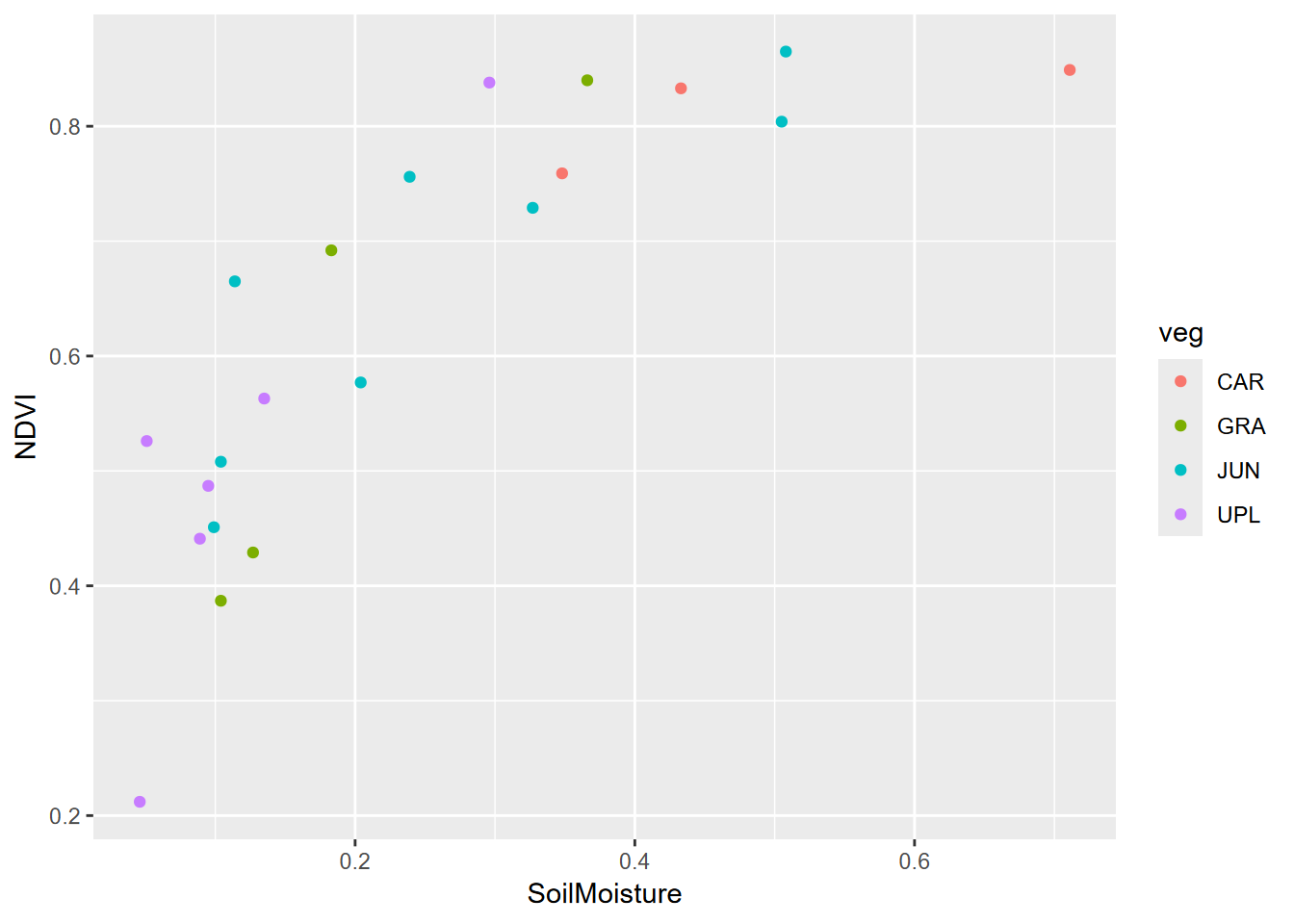Figure 8.10: An identical raster distance output created using a template raster

Note that if you knew of a reference raster, you can also look at its properties with

elev
## class       : SpatRaster
## dimensions  : 134, 167, 1  (nrow, ncol, nlyr)
## resolution  : 30, 30  (x, y)
## extent      : 481905, 486915, 4599195, 4603215  (xmin, xmax, ymin, ymax)
## coord. ref. : NAD_1983_UTM_Zone_10N (EPSG:26910)
## source      : elev.tif
## name        : elev
## min value   : 1336
## max value   : 2260

and use or modify those properties to manually create a template raster, with code something like:

templateRas <- rast(ncol=167, nrow=134,
xmin=481905, xmax=486915, ymin=4599195, ymax=4603215,
vals=1, crs="EPSG:26910")

The purpose here is just to show how we can create and use a raster template. How you define your raster template will depend on the extent and scale of your data, and if you have other rasters (like elev above) you want to match with, you may want to use it as a template as we did first.

Then in deriving distances, it’s useful to remember that distances can go on forever (well, on the planet they may go around and around, if we were using spherical coordinates) so that’s another reason we have to specify the raster structure we want to populate.

## 8.4 Extracting values

A very useful method or environmental analysis and modeling is to extract values from rasters at specific point locations. The point locations might be observations of species, soil samples, or even random points, and getting continuous (or discrete) raster observations can be very useful in a statistical analysis associated with those points. The distance from streams raster we derived earlier, or elevation, or terrain derivatives like slope and aspect might be very useful in a ecological niche model, for instance. We’ll start by using random points and use these to extract values from 4 rasters:

• elev: read in from elev.tif
• slope: created from elev with terrain
• str_dist: Euclidean distance to streams
• geol: rasterized from geology polygon features
library(igisci); library(terra)
geolshp <- vect(ex("marbles/geology.shp"))
streams <- vect(ex("marbles/streams.shp"))
elev <- rast(ex("marbles/elev.tif"))
slope <- terrain(elev, v="slope")
str_dist <- distance(rasterize(streams,elev))
geol <- rasterize(geolshp,elev,field="CLASS")

Note that in contrast to the other rasters, the stream distance raster ends up with no name, so we should give it a name:

names(slope)
##  "slope"
names(str_dist)
##  ""
names(str_dist) <- "str_dist"

On the raster names property: You’ll find that many terra functions may not assign the names property you’d expect, so it’s a good idea to check with names() and maybe set it to what we want, as we’ve just done for str_dist. As we’ll see later with the focal statistics function, the name of the input is used even though we’ve modified it in the function result, and that may create confusion when we use it. We just saw that the distance() function produced an empty name, and there may be others you’ll run into. For many downstream uses, the names property may not matter, but it will be important when we extract values from rasters into points where the names property is assigned to the variable created for the points.

Then we’ll create 200 random xy points within the extent of elev, and assign it the same crs.

library(sf)
x <- runif(200, min=xmin(elev), max=xmax(elev))
y <- runif(200, min=ymin(elev), max=ymax(elev))
rsamps <- st_as_sf(data.frame(x,y), coords = c("x","y"), crs=crs(elev))

To visualize where the random points land, we’ll map them on the geology sf, streams, and contours created from elev using default settings. The terra::as.contour function will create these as SpatVector data, which along with streams we’ll convert with sf::st_as_sf to display in ggplot:

library(tidyverse)
cont <- st_as_sf(as.contour(elev, nlevels=30))
geology <- st_read(ex("marbles/geology.shp"))
ggplot() +
geom_sf(data=geology, aes(fill=CLASS)) +
geom_sf(data=cont, col="gray") +
geom_sf(data=rsamps) +
geom_sf(data=st_as_sf(streams), col="blue")Figure 8.11: Random points in Marble Mountains

Now we’ll extract data from each of the rasters, using an S4 version of rsamps, and then bind them together with the rsamps sf. and . We’ll have to use terra::vect and sf::st_as_sf to convert feature data to the type required by specific tools, and due to a function naming issue, we’ll need to use the package prefix with terra::extract, but otherwise the code is pretty straightforward.

rsampS4 <- vect(rsamps)
elev_ex <- terra::extract(elev, rsampS4) %>% dplyr::select(-ID)
slope_ex <- terra::extract(slope, rsampS4) %>% dplyr::select(-ID)
geol_ex <- terra::extract(geol, rsampS4) %>% dplyr::rename(geology = CLASS) %>% dplyr::select(-ID)
strD_ex <- terra::extract(str_dist, rsampS4)  %>% dplyr::select(-ID)
rsampsData <- bind_cols(rsamps, elev_ex, slope_ex, geol_ex, strD_ex)

Then plot the map with the points colored by geology…

ggplot() +
geom_sf(data=cont, col="gray") +
geom_sf(data=rsampsData, aes(col=geology)) +
geom_sf(data=st_as_sf(streams), col="blue")Figure 8.12: Points colored by geology extracted from raster

… and finally str_dist by elev, colored by geology, derived by extracting. Of course other analyses and visualizations are possible.

ggplot(data=rsampsData, aes(x=str_dist,y=elev,col=geology)) +
geom_point() + geom_smooth(method = "lm", se=F)Figure 8.13: Elevation by stream distance, colored by geology, random point extraction

Here’s a similar example, but using water sample data, which we can then use to relate to extracted raster values to look at relationships. It’s worthwhile to check various results along the way, as we did above. Most of the code is very similar to what we used above, including dealing with naming the distance rasters.

streams <- vect(ex("marbles/streams.shp"))
trails <- vect(ex("marbles/trails.shp"))
elev <- rast(ex("marbles/elev.tif"))
geolshp <- vect(ex("marbles/geology.shp"))
dplyr::select(CATOT, MGTOT, PH, TEMP, TDS)
samples <- vect(sampsf)
strms <- rasterize(streams,elev)
tr <- rasterize(trails,elev)
geol <- rasterize(geolshp,elev,field="CLASS")
stdist <- distance(strms); names(stdist) <- "stDist"
trdist <- distance(tr); names(trdist) = "trDist"
slope <- terrain(elev, v="slope")
aspect <- terrain(elev, v="aspect")
elev_ex <- terra::extract(elev, samples) %>% dplyr::select(-ID)
slope_ex <- terra::extract(slope, samples) %>% dplyr::select(-ID)
aspect_ex <- terra::extract(aspect, samples) %>% dplyr::select(-ID)
geol_ex <- terra::extract(geol, samples) %>% dplyr::rename(geology = CLASS) %>% dplyr::select(-ID)
strD_ex <- terra::extract(stdist, samples) %>% dplyr::select(-ID)
trailD_ex <- terra::extract(trdist, samples) %>% dplyr::select(-ID)
samplePts <- cbind(samples,elev_ex,slope_ex,aspect_ex,geol_ex,strD_ex,trailD_ex)
samplePtsDF <- as.data.frame(samplePts)
head(samplePtsDF)
##   CATOT MGTOT   PH TEMP  TDS elev     slope     aspect geology   stDist
## 1  0.80  0.02 7.74 15.3 0.16 1357  1.721006 123.690068     Qal   0.0000
## 2  0.83  0.04 7.88 14.8 0.16 1359  6.926249 337.833654     Qal  30.0000
## 3  0.83  0.04 7.47 15.1 0.12 1361  4.222687 106.389540     Qal   0.0000
## 4  0.63  0.03 7.54 15.8 0.17 1356  2.160789 353.659808     Qal   0.0000
## 5  0.67  0.06 7.67 14.0 0.14 1374  1.687605  81.869898     Qal   0.0000
## 6  0.70  0.04 7.35  7.0 0.16 1399 12.713997   4.236395    msvu 192.0937
##     trDist
## 1 169.7056
## 2 318.9044
## 3 108.1665
## 4 241.8677
## 5 150.0000
## 6 342.0526
ggplot(data=samplePtsDF, aes(x=geology,y=CATOT)) + geom_boxplot()Figure 8.14: Dissolved calcium carbonate grouped by geology extracted at water sample points

ggplot(data=samplePtsDF, aes(x=slope,y=elev,col=geology)) + geom_point()Figure 8.15: Slope by elevation colored by extracted geology

cont <- st_as_sf(as.contour(elev, nlevels=30))
ggplot() +
geom_sf(data=st_as_sf(geolshp), aes(fill=CLASS)) +
geom_sf(data=cont, col="gray") +
geom_sf(data=st_as_sf(streams), col="blue") +
geom_sf(data=sampsf, aes(size=log(CATOT)))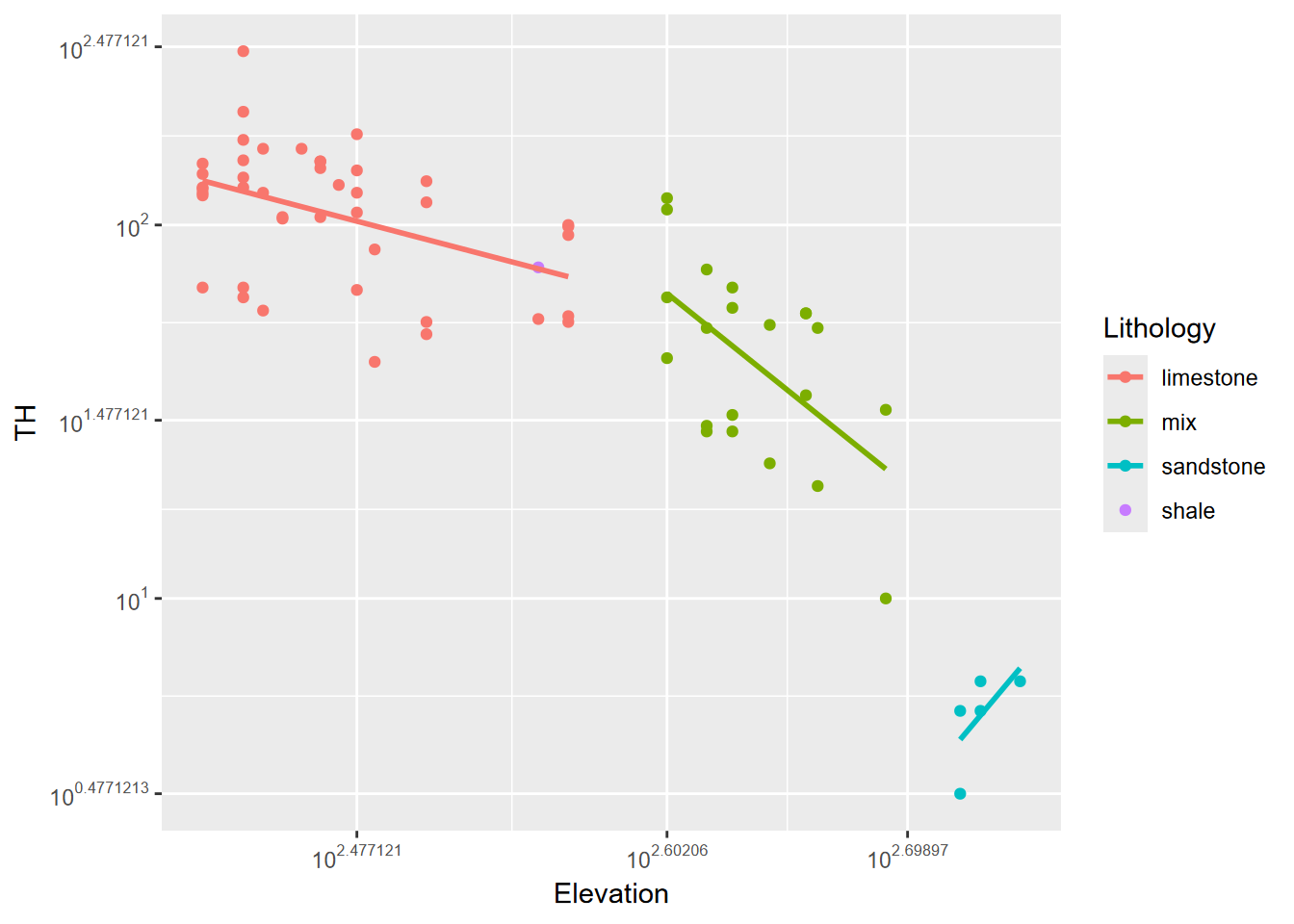Figure 8.16: Log(CATOT) at sample points, showing geologic units

## 8.5 Focal statistics

Focal (or neighborhood) work with a continuous or categorical raster to pass a moving window through it, assigning the central cell with summary statistic applied to the neighborhood, which by default is a 3x3 neighborhood (w=3) centered on the cell. One of the simplest is a low-pass filter where fun="mean". This applied to a continuous raster like elevation will look very similar to the original, so we’ll apply a larger 9x9 (w=9) window so we can see the effect:

plot(elev)Figure 8.17: Elevation

elevLowPass9 <- terra::focal(elev,w=9,fun="mean")
names(elevLowPass9) <- "elevLowPass9" # otherwise gets "elev"
plot(elevLowPass9)Figure 8.18: 9x9 focal mean of elevation

The effect is probably much more apparent in a hillshade, where the very smooth 9x9 low-pass filtered elevation will seem to create an out-of-focus hillshade.

slope9 <- terrain(elevLowPass9, v="slope")
aspect9 <- terrain(elevLowPass9, v="aspect")
plot(shade(slope9/180*pi, aspect9/180*pi, angle=40, direction=330), col=gray(0:100/100))Figure 8.19: Hillshade of 9x9 focal mean of elevation

For categorical/factor data, the modal class in the neighborhood can be defined.

plot(geol)Figure 8.20: Marble Mountains geology raster

plot(terra::focal(geol,w=9,fun="modal"))Figure 8.21: Modal geology in 9x9 neighborhoods

Note that while plot displayed these with a continuous legend, the modal result is going to be an integer value representing the modal class, the most common rock type in the neighborhood. This is sometimes called a majority filter. Challenge: how could we link the modes to the original character CLASS value, and produce a more useful map?

## 8.6 Zonal statistics

Zonal statistics let you stratify by zone, and is a lot like the grouped summary we’ve done before, but in this case the groups are connected to the input raster values by location. There’s probably a more elegant way of doing this but here are a few, that are then joined together.

meanElev <- zonal(elev,geol,fun=mean) %>% rename(mean=elev)
maxElev <- zonal(elev,geol,fun=max) %>% rename(max=elev)
minElev <- zonal(elev,geol,fun=min) %>% rename(min=elev)
left_join(left_join(meanElev,maxElev,by="CLASS"),minElev,by="CLASS")
##   CLASS     mean  max  min
## 1    Ju 1940.050 2049 1815
## 2   Qal 1622.944 1825 1350
## 3   mbl 1837.615 2223 1463
## 4   mmv 1846.186 2260 1683
## 5    ms 1636.802 1724 1535
## 6  msvu 1750.205 2136 1336
## 7    um 1860.758 2049 1719

## 8.7 Interpolation using gstat

A lot of environmental data is captured in point samples, which might be from soil properties, the atmosphere (like weather stations that capture temperature, precipitation, pressure and winds), properties in surface water bodies or aquifers, etc. While it’s often useful to just use those point observations and evaluate spatial patterns of those measurements, we also may want to predict a “surface” of those variables over the map, and that’s where interpolation comes in.

In this section, we’ll look at a few interpolators – nearest neighbor, inverse distance weighting (IDW) and kriging – but the reader should refer to more thorough coverage in Jakub Nowosad’s Geostatistics in R () or at https://rspatial.org. For data, we’ll use the Sierra climate data and derive the annual summaries that we looked at in the last chapter.

We’ll be working in the Teale Albers projection which is good for California, in metres.

Teale<-"+proj=aea +lat_1=34 +lat_2=40.5 +lat_0=0 +lon_0=-120 +x_0=0 +y_0=-4000000 +datum=WGS84 +units=m"
sierra <- st_transform(sierraAnnual, crs=Teale)
counties <- st_transform(CA_counties, crs=Teale)
sierraCounties <- st_transform(sierraCounties, crs=Teale)
ggplot() +
geom_sf(data=counties) +
geom_sf(data=sierraCounties, fill="grey", col="black") +
geom_sf(data=sierra, aes(col=PRECIPITATION))Figure 8.22: Precipitation map in Teale Albers in Sierra counties

### 8.7.1 RMSE of the original data

We’ll start by creating a null model of the z intercept, z being precipitation (x & y being location), as the root mean squared error (RMSE) of individual precipitation values as compared to the mean. The RMSE or rmse function is part of the Metrics package which is loaded with base R, and is simply the square root of the mean squared deviation from the mean.

$RMSE =\sqrt{\sum_{i=1}^{n}{(\bar{z}-z_i)^2}}$

RMSE <- function(observed, predicted) {
sqrt(mean((predicted - observed)^2, na.rm=TRUE))
}
null <- RMSE(mean(sierra$PRECIPITATION), sierra$PRECIPITATION)
null
##  460.6205

### 8.7.2 Voronoi polygon

We’re going to develop a simple prediction using Voronoi polygons (), so we’ll create these and see what they look like with the original data points also displayed. Note that we’ll need to convert to S4 objects using vect():

library(terra)
# proximity (Voronoi/Thiessen) polygons
sierraV <- vect(sierra)
v <- voronoi(sierraV)
plot(v)
points(sierraV)Figure 8.23: Voronoi polygons around Sierra stations

Voronoi polygons keep going outward to some arbitrary limit, so for a more realistic tesselation, we’ll crop out the Sierra counties we selected earlier that contain weather stations. Note that the Voronoi polygons contain all of the attribute data from the original points, just associated with polygon geometry now.

Now we can see a map that displays the original intent envisioned by the meteorologist Thiessen () to apply observations to a polygon area surrounding the station. Voronoi polygons or the raster determined from them (next) will also work with categorical data.

vSierra <- crop(v, vect(st_union(sierraCounties)))
plot(vSierra, "PRECIPITATION")Figure 8.24: Precipitation mapped by Voronoi polygon

Now we’ll rasterize those polygons:

# rasterize
r <- rast(vSierra, res=1000)  # This builds a blank raster of a given dimension and resolution
# We're in Teale metres, so the res is 1000 m
# We'll use this several times below
vr <- rasterize(vSierra, r, "PRECIPITATION")
plot(vr)Figure 8.25: Rasterized Voronoi polygons

Cross validation is used to see how well the model works by sampling it defined number of times (folds) to pull out sets of training and testing samples, and then comparing the model’s predictions with the (unused) testing data. Each time through, the model is built out of that fold’s training data. For each fold, a random selection is created by the sample function. The mean RMSE of all of the folds is the overall result, and provides an idea on how well the interpolation worked. The first model evaluated is the null model where we’re comparing to the mean of the input data.

# n-fold cross validation
n <- 10
set.seed(5132015)
kf <- sample(1:n, size = nrow(sierraV), replace=TRUE) # get random numbers
# between 1 and 5 to same size as input data points SpatVector sierraV
rmse <- rep(NA, n) # blank set of 5 NAs
for (k in 1:n) {
test <- sierraV[kf == k, ]  # split into 5 sets of points, 4/5 going to training set,
train <- sierraV[kf != k, ] #  and 1/5 to test, including all of the variables
v <- voronoi(train)
v
test
p <- terra::extract(v, test) # extract values from v (built from training data) at test locations
rmse[k] <- RMSE(test$PRECIPITATION, p$PRECIPITATION)
}
rmse
##    288.4298  266.3894  192.2506  104.9504  261.9054  465.7811  203.9854
##    260.4066 1011.7092  519.7488
mean(rmse)
##  357.5557
# relative model performance
perf <- 1 - (mean(rmse) / null)
round(perf, 3)
##  0.224

### 8.7.3 Nearest neighbor interpolation

The previous assignment of data to Voronoi polygons can be considered to be a nearest neighbor interpolation where only one neighbor is used, but we can instead use multiple neighbors. In this case we’ll use up to 5 (nmax=5). Presumably setting idp to zero makes it a nearest neighbor, along with the nmax setting.

library(gstat)
d <- data.frame(geom(sierraV)[,c("x", "y")], as.data.frame(sierraV))
head(d)
##           x          y        STATION_NAME  LONGITUDE LATITUDE ELEVATION
## 1 105093.88 -168859.68        ASH MOUNTAIN  -118.8253  36.4914     520.6
## 2  43245.02 -102656.13        AUBERRY 2 NW  -119.5128  37.0919       637
## 3  81116.40 -122688.39   BALCH POWER HOUSE -119.08833 36.90917     524.3
## 4  67185.02  -89772.17      BIG CREEK PH 1 -119.24194 37.20639    1486.8
## 5 145198.34  -70472.98      BISHOP AIRPORT -118.35806 37.37111    1250.3
## 6 -61218.92  140398.66 BLUE CANYON AIRPORT  -120.7102  39.2774    1608.1
##   PRECIPITATION TEMPERATURE
## 1        688.59    17.02500
## 2        666.76    16.05833
## 3        794.02    16.70000
## 4        860.05    11.73333
## 5        131.58    13.61667
## 6       1641.34    10.95000
gs <- gstat(formula=PRECIPITATION~1, locations=~x+y, data=d, nmax=5, set=list(idp = 0))
nn <- interpolate(r, gs, debug.level=0)
plot(nnmsk, 1)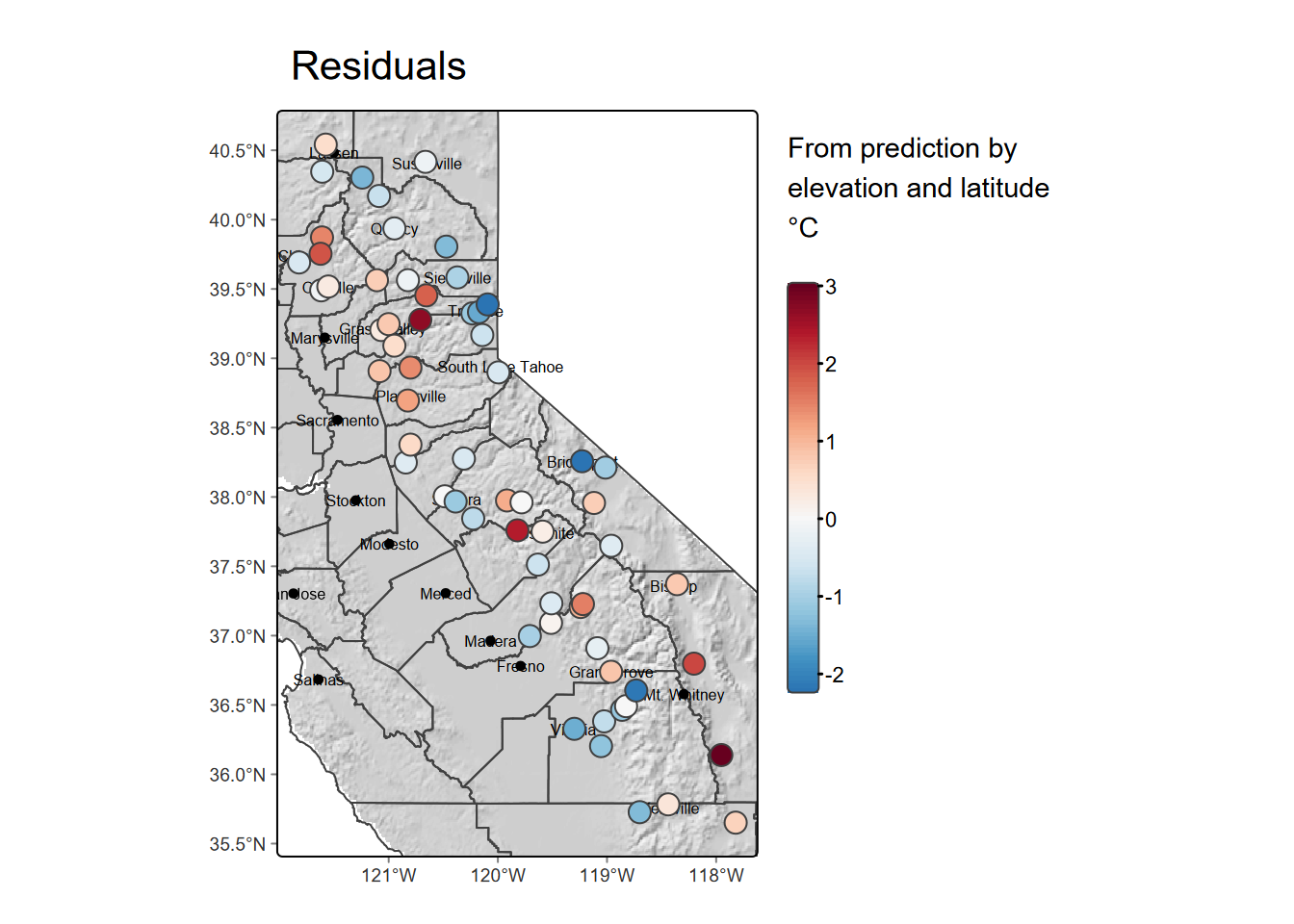Figure 8.26: Nearest neighbor interpolation of precipitation

Cross validation for our nearest neighbor model with nmax=5 and relative performance:

rmsenn <- rep(NA, n)
for (k in 1:n) {
test <- d[kf == k, ]
train <- d[kf != k, ]
gscv <- gstat(formula=PRECIPITATION~1, locations=~x+y, data=train, nmax=5, set=list(idp = 0))
p <- predict(gscv, test, debug.level=0)$var1.pred rmsenn[k] <- RMSE(test$PRECIPITATION, p)
}
rmsenn
##   337.5685 374.0160 176.6017 477.0555 219.7810 508.0988 284.8718 303.7919
##   752.4552 352.6487
mean(rmsenn)
##  378.6889
1 - (mean(rmsenn) / null)
##  0.1778723

The higher RMSE and lower relative performance compared with the Voronoi model suggests that it’s not any better than that single-neighbor model, so we might just stick with that.

### 8.7.4 Inverse Distance Weighted (IDW)

The Inverse Distance Weighted (IDW) interpolator is popular due to its ease of use and few statistical assumptions. Surrounding points influence the predicted value of a cell based on the inverse of their distance. The inverse distance weight has an an exponent set with set=list(idp=2), and if that is 2 (so inverse distance squared), it is sometimes referred to as a “gravity model” since it’s the same as the way gravity works. For environmental variables however sampling locations are not like objects that create an effect but rather observations of a continuous surface. A power of 1 reduces the planet-like emphasis on the sampling point, so we’ll use that.

library(gstat)
gs <- gstat(formula=PRECIPITATION~1, locations=~x+y, data=d, nmax=Inf, set=list(idp=3))
idw <- interpolate(r, gs, debug.level=0)
plot(idwr, 1)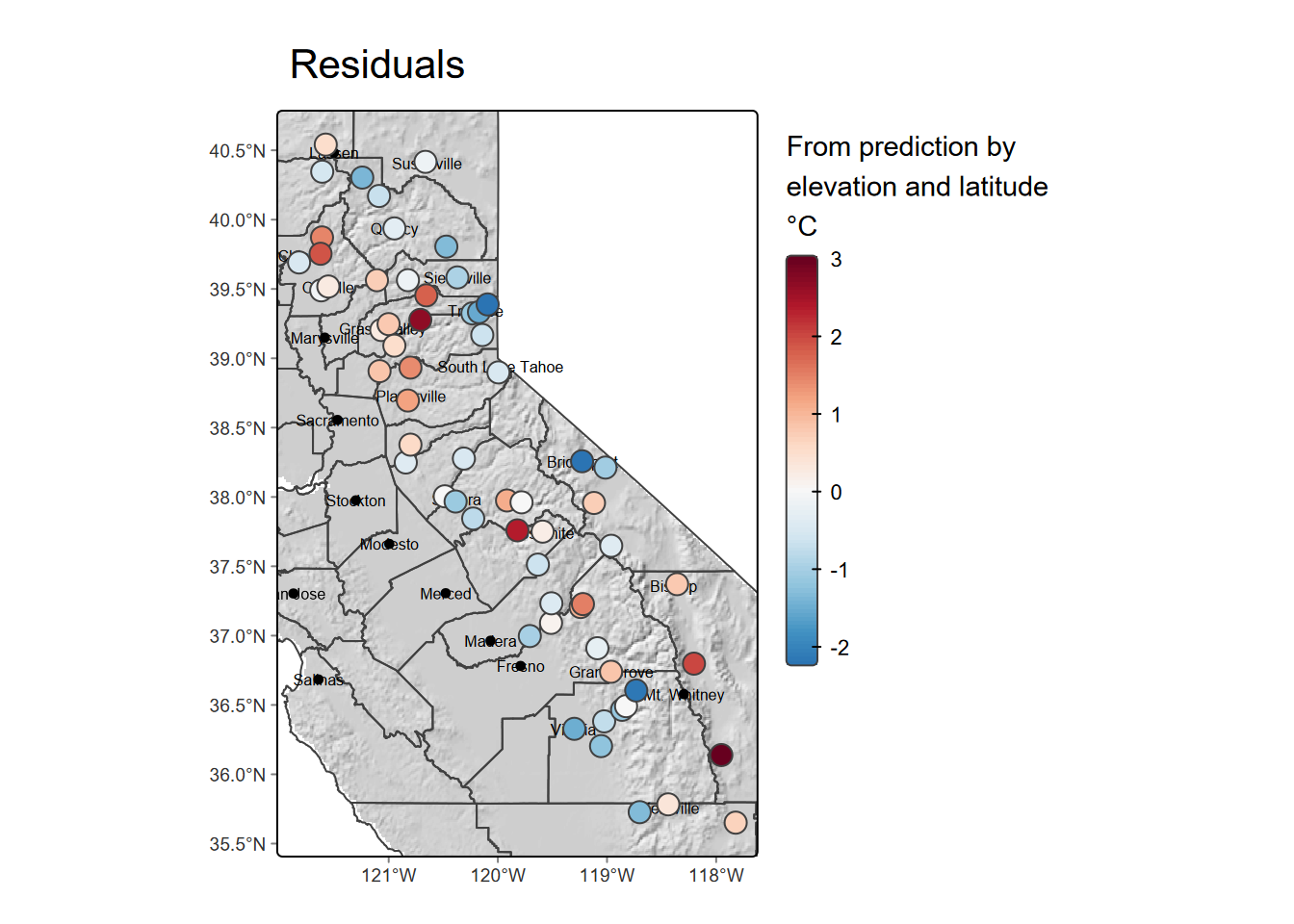Figure 8.27: IDW interpolation, power=2

Cross validate IDW and relative performance:

rmse <- rep(NA, n)
for (k in 1:n) {
test <- d[kf == k, ]
train <- d[kf != k, ]
gs <- gstat(formula=PRECIPITATION~1, locations=~x+y, data=train, set=list(idp=3))
p <- predict(gs, test, debug.level=0)
rmse[k] <- RMSE(test$PRECIPITATION, p$var1.pred)
}
rmse
##   292.4043 266.1711 174.0900 347.6625 221.8734 472.8975 170.6611 327.5149
##   650.4894 351.5487
mean(rmse)
##  327.5313
1 - (mean(rmse) / null)
##  0.2889347

So what we can see from the above is the IDW has a bit better relative performance (lower RMSE). The IDW does come with an artifact of pits and peaks around the data points, which can be decreased with a lower idp. We’ll try a square root (idp=0.5):

library(gstat)
gs <- gstat(formula=PRECIPITATION~1, locations=~x+y, data=d, nmax=Inf, set=list(idp=0.5))
idw <- interpolate(r, gs, debug.level=0)
plot(idwr, 1)Figure 8.28: IDW interpolation, power=0.5

rmse <- rep(NA, 5)
for (k in 1:5) {
test <- d[kf == k, ]
train <- d[kf != k, ]
gs <- gstat(formula=PRECIPITATION~1, locations=~x+y, data=train, set=list(idp=0.5))
p <- predict(gs, test, debug.level=0)
rmse[k] <- RMSE(test$PRECIPITATION, p$var1.pred)
}
rmse
##  498.7192 270.5047 435.7024 159.4544 344.1800
mean(rmse)
##  341.7121
1 - (mean(rmse) / null)
##  0.2581483

… but the relative performance is low.

### 8.7.5 Polynomials and trend surfaces

In the Voronoi, nearest-neighbor and IDW methods above, predicted cell values were based on values of nearby input points, in the case of the Voronoi creating a polygon surrounding the input point to assign its value to (and the raster simply being those polygons assigned to raster cells.) Another approach is to create a polynomial model where values are assigned based on their location defined as x and y values. The simplest is first-order linear trend surface model, as the equation of a plane:

$z = b_0 + b_1x + b_2y$

where z is a variable like PRECIPITATION. Higher order polynomials can model more complex surfaces such as a fold using a second order polynomial which would look like:

$z = b_0 + b_1x + b_2y + b_3x^2 + b_4xy + b_5y^2$

… or a third-order polynomial which might model a dome or basin:

$z = b_0 + b_1x + b_2y + b_3x^2 + b_4xy + b_5y^2 + b_6x^3 + b_7x^2y + b_8xy^2 + b_9y^3$

To enter the first order, your formula can look like z~x+y but anything beyond this pretty much requires using the degree setting. For the first order this would include z~1 and degree=1; the second order just requires changing the degree to 2, etc.

We’ll use gstat with a global linear trend surface by providing this formula as TEMPERATURE~x+y, and use the degree setting:

library(gstat)
gs <- gstat(formula=PRECIPITATION~1, locations=~x+y, degree=1, data=d)
interp <- interpolate(r, gs, debug.level=0)
plot(prec1, 1)Figure 8.29: Linear trend

What we’re seeing is a map of a sloping plane showing the general trend. Not surprisingly there are areas with values lower than the minimum input temperature and higher than the maximum input temperature, which we can check with:

min(d$PRECIPITATION) ##  125.48 max(d$PRECIPITATION)
##  2103.6

… but that’s what we should expect when defining a trend that extends beyond our input point locations.

Then we’ll try a 2nd order:

gs <- gstat(formula=PRECIPITATION~1, locations=~x+y, degree=2, data=d)
interp2 <- interpolate(r, gs, debug.level=0)
plot(prec2, 1)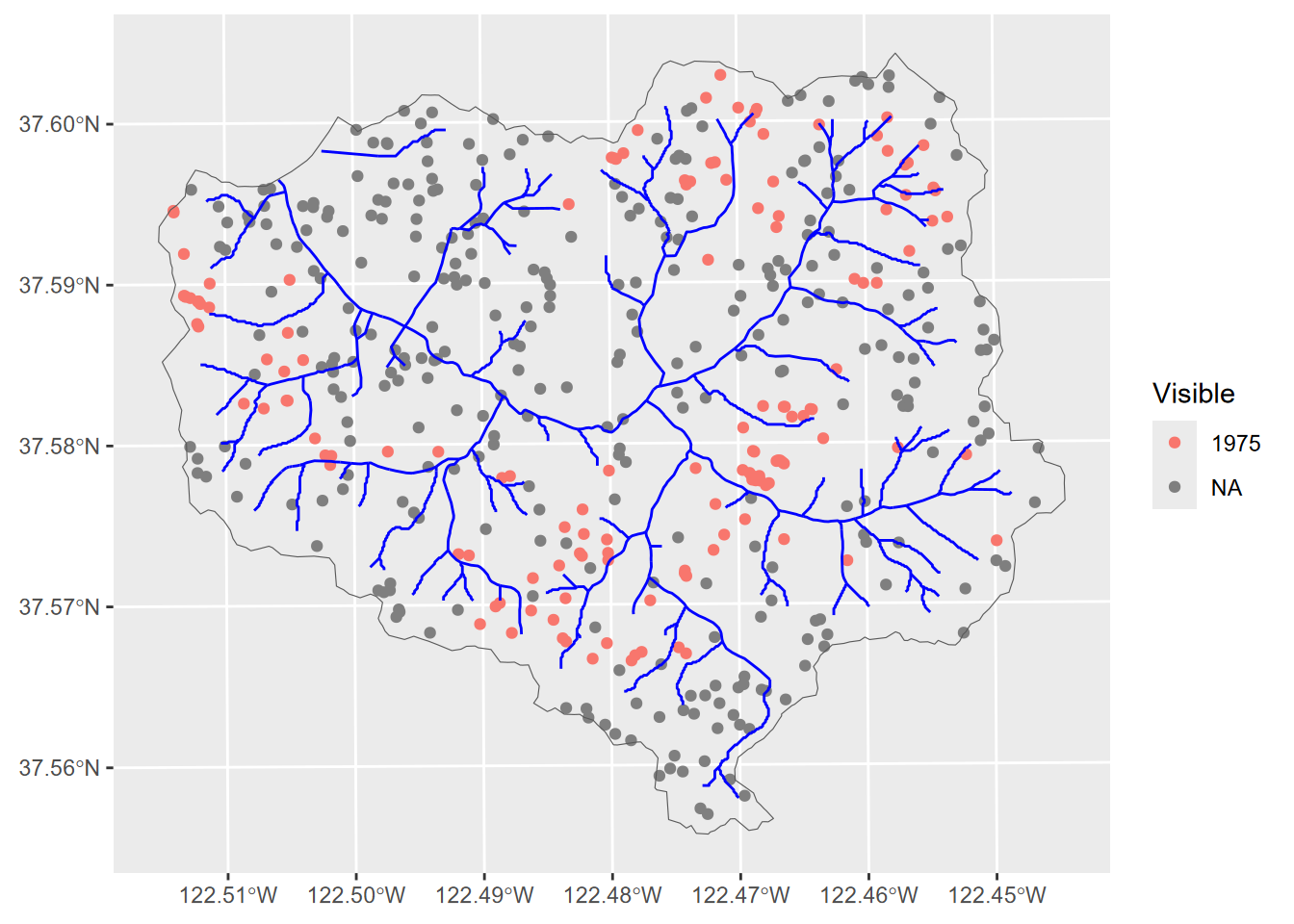Figure 8.30: 2nd order polynomial, precipitation

… which exhibits this phenomenon all the more, though only away from our input data. Then a 3rd order …

gs <- gstat(formula=PRECIPITATION~1, locations=~x+y, degree=3, data=d)
interp3 <- interpolate(r, gs, debug.level=0)
plot(prec3, 1)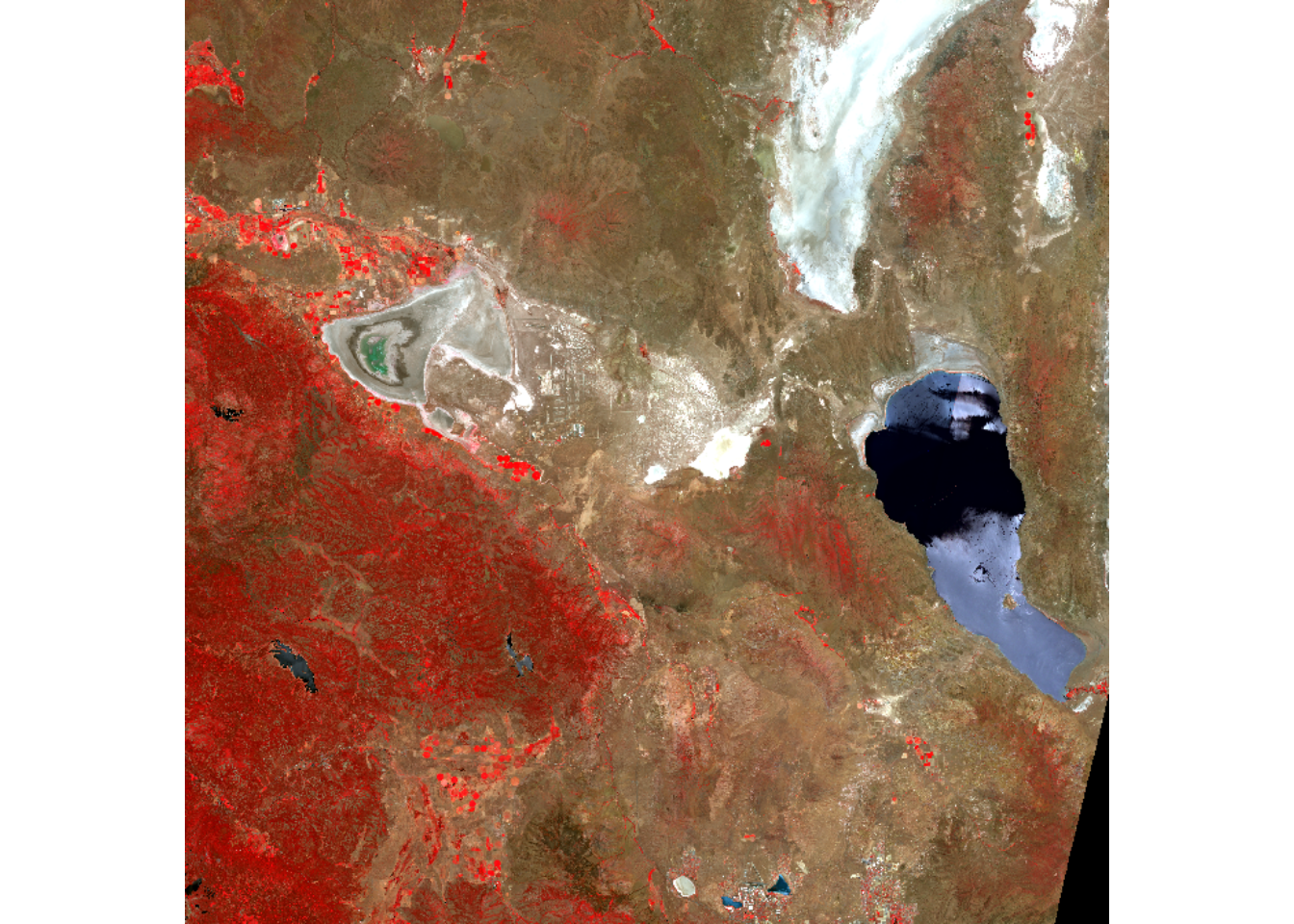Figure 8.31: 3rd order polynomial, temperature

… extends this to the extreme, though the effect is only away from our data points so it’s just a cartographic problem we could address by controlling the range of values over which to apply the color range. Or we could use map algebra to assign all cells higher than the maximum inputs to the maximum and lower than the minimum inputs to the minimum:

prec3$var1.pred[prec3$var1.pred > max(d$PRECIPITATION)] <- max(d$PRECIPITATION)
prec3$var1.pred[prec3$var1.pred < min(d$PRECIPITATION)] <- min(d$PRECIPITATION)
plot(prec3$var1.pred)Figure 8.32: 3rd order polynomial with extremes flattened Then a 3rd order local polynomial (setting nmax to less than Inf), where we’ll similarly set the values outside the range to the min or max value. The nmax setting can be seen to have a significant effect on the smoothness of the result. gs <- gstat(formula=PRECIPITATION~1, locations=~x+y, degree=3, nmax=30, data=d) interp3loc <- interpolate(r, gs, debug.level=0) prec3loc <- mask(interp3loc, vr) prec3loc$var1.pred[prec3loc$var1.pred > max(d$PRECIPITATION)] <- max(d$PRECIPITATION) prec3loc$var1.pred[prec3loc$var1.pred < min(d$PRECIPITATION)] <- min(d$PRECIPITATION) plot(prec3loc$var1.pred)Figure 8.33: 3rd order polynomial, precipitation

### 8.7.6 Kriging

Kriging requires a lot of exploratory work with visual interpretation of data to derive the right model parameters. It starts by looking at the variogram and creating a model with parameters to see which might best approximates the distribution.

#### 8.7.6.1 Create a variogram.

Compare every point to every other point. The width setting (20,000 m) is the subsequent distance intervals into which data point pairs are grouped for semivariance estimates.

#p <- data.frame(geom(dtakm)[, c("x","y")], as.data.frame(dta))
gs <- gstat(formula=PRECIPITATION~1, locations=~x+y, data=d)
v <- variogram(gs, width=20e3)
v
##     np      dist     gamma dir.hor dir.ver   id
## 1   23  14256.47  45143.82       0       0 var1
## 2   60  30035.37  61133.79       0       0 var1
## 3   93  50919.48 163622.47       0       0 var1
## 4   97  71033.67 192267.26       0       0 var1
## 5  126  89759.82 186047.23       0       0 var1
## 6  109 109139.47 192847.68       0       0 var1
## 7  109 130125.18 139241.93       0       0 var1
## 8   91 149596.56 146620.61       0       0 var1
## 9   94 169606.82 150855.26       0       0 var1
## 10  90 188727.78 203683.08       0       0 var1
## 11  57 208856.39 220665.36       0       0 var1
plot(v)Figure 8.34: Variogram of precipitation at Sierra weather stations

#### 8.7.6.2 Fit the variogram based on visual interpretation

fve <- fit.variogram(v, vgm(psill=2e5, model="Exp", range=200e3, nugget=0))
plot(variogramLine(fve, maxdist=2e5), type='l', ylim=c(0,2e5))
points(v[,2:3], pch=20, col='red')Figure 8.35: Fitted variogram

There are multiple models that can be used. We just used "Exp" (exponential), so now we’ll try "Sph" (spherical). Others are "Gau", "Mat", and the list of 20 can be provided with vgm():

vgm()
##    short                                      long
## 1    Nug                              Nug (nugget)
## 2    Exp                         Exp (exponential)
## 3    Sph                           Sph (spherical)
## 4    Gau                            Gau (gaussian)
## 5    Exc        Exclass (Exponential class/stable)
## 6    Mat                              Mat (Matern)
## 7    Ste Mat (Matern, M. Stein's parameterization)
## 8    Cir                            Cir (circular)
## 9    Lin                              Lin (linear)
## 10   Bes                              Bes (bessel)
## 11   Pen                      Pen (pentaspherical)
## 12   Per                            Per (periodic)
## 13   Wav                                Wav (wave)
## 14   Hol                                Hol (hole)
## 15   Log                         Log (logarithmic)
## 16   Pow                               Pow (power)
## 17   Spl                              Spl (spline)
## 18   Leg                            Leg (Legendre)
## 19   Err                   Err (Measurement error)
## 20   Int                           Int (Intercept)
fvs <- fit.variogram(v, vgm(psill=2e5, model="Sph", range=20e3, nugget=0)) # other models: "Exp",
fvs
##   model    psill    range
## 1   Nug      0.0     0.00
## 2   Sph 183011.2 90689.21
plot(variogramLine(fvs, maxdist=2e5), type='l', ylim=c(0,2e5))
points(v[,2:3], pch=20, col='red')Figure 8.36: Spherical fit

The exponential model at least visually looks like the best fit, so we’ll use the fse model we created first.

plot(v, fve)Figure 8.37: Exponential model

#### 8.7.6.3 Ordinary Kriging

So we’ll go with the fve model derived using an exponential form to develop an ordinary Kriging prediction and variance result. The prediction is the interpolation, and the variance shows the areas near the data points where we should have more confidence in the prediction; this ability is one of the hallmarks of the Kriging method.

k <- gstat(formula=PRECIPITATION~1, locations=~x+y, data=d, model=fve)
kp <- interpolate(r, k, debug.level=0)
names(ok) <- c('prediction', 'variance')
plot(ok)Figure 8.38: Ordinary Kriging

Clearly the prediction provides a clear pattern of precipitation, at least for the areas with low variance. The next step would be to do a cross validation to compare its RMSE with IDW and other models, but we’ll leave that for later.

To learn more about these interpolation methods and related geostatistical methods, see http://rspatial.org/terra/analysis/4-interpolation.html and the gstat user manual at http://www.gstat.org/gstat.pdf .

## 8.8 Exploring Further

There’s always a lot more you can learn about methods than what a book like this will present, and as you’re using R for your own research you’ll find that you need to explore other tools. The help system and additional resources on the packages we’ve started looking at will have more ideas. We’ll also look at some code that includes a variety of methods we’ve learned about in this section.

### 8.8.1 Exploring other analysis functions in sf and terra

Both packages have quite a lot of useful functions, in both raster and vector domains, as you can see in the Help with ?terra. I’d encourage you to explore these, and and are good resources for learning more.

terra : You can use the rasterVis::gplot function to plot a raster in ggplot, since that gplot is a wrapper around ggplot, but I haven’t figured out yet how to also plot vectors on top. So this works just for rasters …

library(rasterVis)
elev <- rast(ex("marbles/elev.tif"))
gplot(elev) + geom_tile(aes(fill=value))

See Appendix A (A8.1) to see an example of a study using raster methods on a karst solution process study in Sinking Cove, Tennessee.

## 8.9 Exercises

1. You can get the four values that define the extent of a raster with terra functions xmin, xmax, ymin, and ymax. Use these with the raster elev created from "marbles/elev.tif", then derive 100 uniform random x and y values with those min and max values. Use cbind to display a matrix of 100 coordinate pairs.

2. Create sf points from these 100 uniform random coordinate pairs. Use tmap to display them on a base of the elevation raster. Your resulting map should look similar to the following (with different random numbers).

1. Now use those points to extract values from stream distance, trail distance, geology, slope, aspect, and elevation, and display that sf data frame as a table, then plot trail distance (x) vs stream distance (y) colored by geology and sized by elevation. Your goal for the table and graph are shown here:Figure 8.39: Goal of Question 3

1. Create a slope raster from “SanPedro/dem.tif” then a “steep” raster of all slopes > 26 degrees, determined by a study of landslides to be a common landslide threshold, then display them using (palette=“Reds,” alpha=0.5, legend.show=F) along with roads “SanPedro/roads.shp” in “black,” streams “SanPedro/streams.shp” in “blue,” and watershed borders “SanPedro/SPCWatershed.shp” in “darkgreen” with lwd=2: